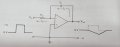# Why Vout is not 0 V in integrator circuit? ?

#### Heroz

Joined May 29, 2022
31
In the circuit below :I understand when Vin is +V , the slope of Vout is straight line .
But I don't understand when Vin is 0 V why V out is not 0 V because when Vin is 0 and there are virtual ground it mean there are voltage drop across Rin 0 V
therefore I in = IF = 0 A

And in equation IF = -C(dVout/dt)

The slope of Vout should be 0 when Vin is 0 V but in picture it's slope . What's wrong in my analysis ?

Thank you.

#### WBahn

Joined Mar 31, 2012
28,193
What is Vout if Vin = 0 V and the voltage across the capacitor is 1.23 V?

#### crutschow

Joined Mar 14, 2008
31,585
The picture is wrong.
When the input voltage is zero, there is no current to charge/discharge the capacitor.
Thus the output voltage stays at whatever value it was prior to the input going to zero.

Also for a positive input, the current directions shown are for electron flow.

•ronsimpson

#### Heroz

Joined May 29, 2022
31
What is Vout if Vin = 0 V and the voltage across the capacitor is 1.23 V?
I read this in my textbook they explained how this circuit work here explanation:
Iin = V in / Rin
I in = IF

When Vin is positive , slope will be as picture above untill capacitor is full and when Vin is 0 V , capacitor will discharge and slope will be as picture above . Does this circuit work and generate triangle signal only when Vin is not 0 V or clock ?

#### WBahn

Joined Mar 31, 2012
28,193
I read this in my textbook they explained how this circuit work here explanation:
Iin = V in / Rin
I in = IF

When Vin is positive , slope will be as picture above untill capacitor is full and when Vin is 0 V , capacitor will discharge and slope will be as picture above . Does this circuit work and generate triangle signal only when Vin is not 0 V or clock ?
When Vin is zero, the current in the resistor is zero, which means that the current in the capacitor is zero, which means that the charge on the capacitor will not change -- it will stay what ever it is.

#### crutschow

Joined Mar 14, 2008
31,585
Last edited:
•Mafenspop

#### Mafenspop

Joined Sep 13, 2014
2
In the circuit below :

View attachment 280245

I understand when Vin is +V , the slope of Vout is straight line .
But I don't understand when Vin is 0 V why V out is not 0 V because when Vin is 0 and there are virtual ground it mean there are voltage drop across Rin 0 V
therefore I in = IF = 0 A

And in equation IF = -C(dVout/dt)

The slope of Vout should be 0 when Vin is 0 V but in picture it's slope . What's wrong in my analysis ?

Thank you.

#### Mafenspop

Joined Sep 13, 2014
2
Is your opamp model ideal, or are the real input parameters included. If so, maybe current leakage is discharging the cap. Otherwise I think Vout should be constant (zero slope).